# Polygons - 11-gons

Properties of 11-gons, interior angles of 11-gons

Polygons:  Properties of 11-gons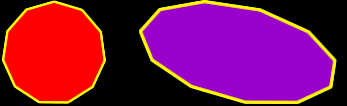Sum of the Interior Angles of an 11-gon:
 This image shows the process for a HEXAGON: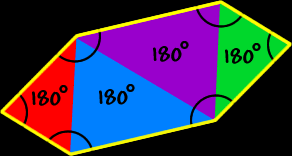Using the same methods as for hexagons to the right (I'll let you do the pictures)... To find the sum of the interior angles of an 11-gon, divide it up into triangles... There are nine triangles...  Because the sum of the angles of each triangle is 180 degrees...  We get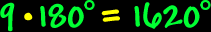So, the sum of the interior angles of an 11-gon is 1620 degrees.Regular 11-gons:
The properties of regular 11-gons: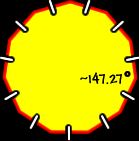All sides are the same length (congruent) and all interior angles are the same size (congruent).

To find the measure of the angles, we know that the sum of all the angles is 1620 degrees (from above)...  And there are eleven angles...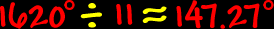So, the measure of the interior angle of a regular 11-gon is about 147.27 degrees.The measure of the central angles of a regular 11-gon: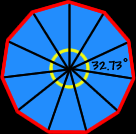To find the measure of the central angle of a regular 11-gon, make a circle in the middle...  A circle is 360 degrees around...  Divide that by eleven angles...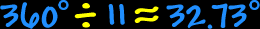So, the measure of the central angle of a regular 11-gon is about 32.73 degrees.